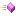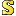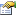In This Topic ▼

Summary

The following tables list the members exposed by LeadMatrix.

Public Constructors

Name DescriptionPublic Methods

Name Descriptionclone Creates an exact copy of this LeadMatrix.create Creates a new LeadMatrix with the specified values.equals Compares two LeadMatrix objects to determine whether they contain the same coordinates.fromJSON Converts a JSON-compatible dictionary object to a new LeadMatrix instance.invert Inverts this LeadMatrix.multiply Multiplies a LeadMatrix by another LeadMatrix.prepend Prepends the specified LeadMatrix onto this LeadMatrix.rotate Applies a rotation of the specified angle about the origin of this LeadMatrix.rotateAt Rotates this matrix about the specified point.rotateAtPrepend Prepends a rotation of the specified angle at the specified point to this LeadMatrix.rotatePrepend Prepends a rotation of the specified angle to this LeadMatrix.scale Appends the specified scale vector to this LeadMatrix.scaleAt Scales this LeadMatrix by the specified amount about the specified point.scaleAtPrepend Prepends the specified scale about the specified point of this LeadMatrix.scalePrepend Prepends the specified scale vector to this LeadMatrix.setIdentity Changes this LeadMatrix into an identity matrix.skew Appends a skew of the specified degrees in the x and y dimensions to this LeadMatrix.skewPrepend Prepends a skew of the specified degrees in the x and y dimensions to this LeadMatrix.toJSON Converts this LeadMatrix to a JSON-compatible dictionary object.toString Converts this LeadMatrix to a human-readable string.transformPoint Transforms the specified point by the LeadMatrix and returns the result.transformPoints Transforms the specified points by this LeadMatrix.transformRect Transforms the specified rect by the LeadMatrix and returns the result.transformVector Transforms the specified vector point by the LeadMatrix and returns the result.translate Appends a translation of the specified offsets to this LeadMatrix.translatePrepend Prepends a translation of the specified offsets to this LeadMatrix.

Public Properties

Name Descriptiondeterminant Gets the determinant of this LeadMatrix.hasInverse Gets a value that indicates whether this LeadMatrix is invertible.identity Gets an identity LeadMatrix.isIdentity Gets a value that indicates whether this LeadMatrix is an identity matrix.m11 Gets or sets the value of the first row and first column of this LeadMatrix.m12 Gets or sets the value of the first row and second column of this LeadMatrix.m21 Gets or sets the value of the second row and first column of this LeadMatrix.m22 Gets or sets the value of the second row and second column of this LeadMatrix.offsetX Gets or sets the value of the third row and first column of this LeadMatrix.offsetY Gets or sets the value of the third row and first column of this LeadMatrix.